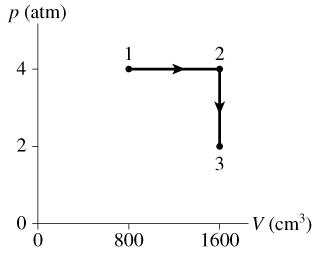# Problem: 0.10 mol of a monatomic gas follows a process shown in the figure.How much energy is transferred to or from the gas during a process from 1 to 2?

###### FREE Expert Solution

The ideal gas equation for real gases:

$\overline{){\mathbf{P}}{\mathbf{V}}{\mathbf{=}}{\mathbf{n}}{\mathbf{R}}{\mathbf{T}}}$, where P is the gas pressure, V is the volume of the gas, n is the number of moles of the gas, R is the gas constant, and T is the absolute gas temperature.

Change in the gas internal energy:

$\overline{){\mathbf{∆}}{{\mathbf{E}}}_{\mathbf{t}\mathbf{h}}{\mathbf{=}}\frac{\mathbf{3}}{\mathbf{2}}{\mathbf{n}}{\mathbf{R}}{\mathbf{∆}}{\mathbf{T}}}$

Work done by the gas:

$\overline{){{\mathbf{W}}}_{\mathbf{g}\mathbf{a}\mathbf{s}}{\mathbf{=}}{\mathbf{P}}{\mathbf{∆}}{\mathbf{V}}}$, where ΔV= V2 - V1.

The temperature of the monoatomic gas at the different points can be found from the ideal gas equation:

At Point 1:

T1 = P1V1/nR

81% (198 ratings)###### Problem Details

0.10 mol of a monatomic gas follows a process shown in the figure.How much energy is transferred to or from the gas during a process from 1 to 2?